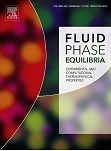# Simple and predictive approach for calculating the high pressure and temperature vapour—liquid equilibria of binary mixtures by applying a UNIFAC equation of state method

Simple and predictive approach for calculating the high pressure and temperature vapour—liquid equilibria of binary mixtures by applying a UNIFAC equation of state method

Santacesaria, E.; Tesser, R.; Di Serio, M.

Fluid Phase Equilibria,84 (1993) 1ll-122

https://doi.org/10.1016/0378-3812(91)80039-X

Abstract

A simple and predictive for describing the high P-T vapour-liquid equilibria (VLE) of binary mixtures is described. It is based on the use of group contribution method such as UNIFAC combined with the Redlich-Kwong-Soave (RKS) equation of state (EOS) and quadratic mixing rules. VLE data are generated by UNIFAC at a prefixed temperature. These data are used for determining by regression analysis the binary interactin parameters of the RKS/EOS. Then the EOS with the same parameters can be used for describing VLE at higher temperatures.